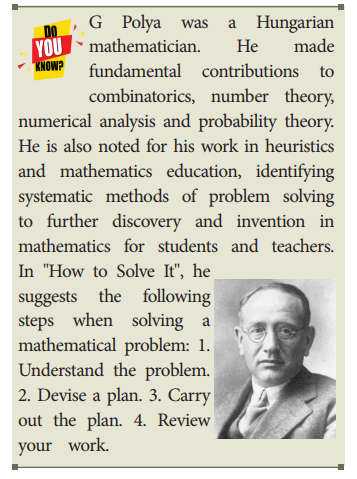# Algorithms

An algorithm is a sequence of instructions to accomplish a task or solve a problem.

Algorithms

An algorithm is a sequence of instructions to accomplish a task or solve a problem. An instruction describes an action. When the instructions are executed, a process evolves, which accomplishes the intended task or solves the given problem. We can compare an algorithm to a recipe, and the resulting process to cooking.

We are interested in executing ouralgorithms in a computer. A computer can only execute instructions in a programming language. Instructions of a computer are also known as statements. Therefore, ultimately, algorithms must be expressed using statements of a programming language.

A problem is specified by given in put data, desired output data and a relation between the input and the output data. An algorithm starts execution with the input data, executes the statements, and finishes execution with the output data. When it finishes execution, the specified relation between the input data and the output data should be satisfied. Only then has the algorithm solved the given problem.

An algorithm is a step by step sequence of statements intended to solve a problem. When executed with input data, it generates a computational process, and ends with output data, satisfying the specified relation between the input data and the output data.

Example 6.1. Add two numbers: To add two numbers, we proceed by adding the right most digits of the two numbers, then the next right most digits together with carry that resulted from the previous (right) position, and so on. The computational process for adding 2586 and 9237 is illustrated in Table 6.1.Tags : Computer Science , 11th Computer Science : Chapter 6 : Specification and Abstraction
Study Material, Lecturing Notes, Assignment, Reference, Wiki description explanation, brief detail
11th Computer Science : Chapter 6 : Specification and Abstraction : Algorithms | Computer Science